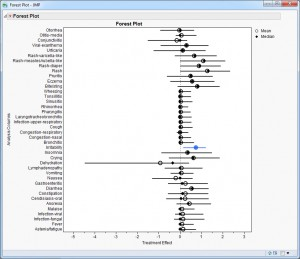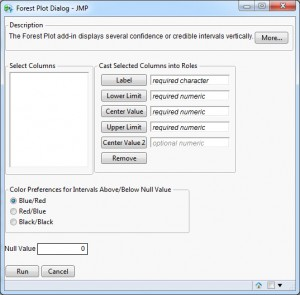Choose Language Hide Translation Bar
Forest Plot Add-In for JMP 10

A forest plot (Figure 1) is a convenient way to graphically display several confidence or credible intervals and is often used in meta-analysis. Here, the x-axis represents the treatment effect between two interventions while the y-axis refers to the individual studies from which the intervals are obtained, or to various consensus intervals derived from the pool of available studies.

This is by no means the only application of these plots. Forest plots are useful to summarize several endpoints from a single clinical trial, say the incidence of adverse events between two treatments. Or they can be used to examine the robustness of an endpoint across numerous subgroups.A Forest Plot Add-In is now available from the JMP File Exchange (free SAS profile needed for download). The dialog for this add-in is presented in Figure 2.

The add-in assumes that a data table contains the final computed estimates (Center Value, such as the mean treatment difference), confidence intervals (Lower and Upper Limits) and labels (which can refer to endpoint, study or subgroup) for display. Intervals are ordered in the figure according to their order in the data table. You can also provide a second center value (Center Value 2) to present additional summary values (e.g., medians) in the figure. Color options allow you to highlight intervals that exclude the null value (default 0), and this color varies according to whether the interval is above or below the null value. Finally, a dotted vertical reference line is drawn at the null value.

I'll describe a few examples. Figure 1 uses data from Mehrotra and Heyse in comparing adverse event rates among two vaccine groups. The estimates and credible intervals are summarized from 20,000 samples of the posterior distribution estimated using MCMC from a Bayesian hierarchical mixture model described in Berry & Berry. The 2.5 and 97.5 percentiles of these samples are presented as the lower and upper limits, respectively. Both the means (circles) and medians (diamonds) of the 20,000 samples are presented in the plot (as Center Value and Center Value 2, respectively). Only irritability appears to show any difference between the two groups.A second example uses data from Chang et al., a non-inferiority trial in subjects chronically infected with hepatitis B virus (HBV). Figure 3 summarizes the virologic, biochemical and serologic secondary endpoints found in Table 3 of this article. While 0 was used as the null value in the add-in, a second reference line was added at -10%, the non-inferiority margin of the primary endpoint. As seen below, all endpoints appear to favor the test drug, though only three show superiority to the active control. Center Value 2 was not used in this example. Had I wanted the interval coloring to reflect the non-inferiority margin, I would have used -10 as the null value and added a second reference line for 0.